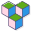CppBuzz# Java program to print table of any number

``````public class Main {

public static void main(String[] args) {
// table of 4 is 4 x 1 = 4
// 4 x 2 = 8
// 4 * 3 = 12

int num = 4; // we can also take input from user

for(int i=1; i<=10; i++) {
System.out.println(num + " x " + i + " = " + (num*i));
}
}
}``````